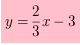### Home > CC4 > Chapter 5 > Lesson 5.3.2 > Problem5-111

5-111.

What is the equation of the line that has a $y$intercept of $(0, –3)$ and passes through the point $(–9, –9)$? 5-111 HW eTool (Desmos). Homework Help ✎

Complete the table in the eTool below to determine the equation of the line.
Click the link at right for the full version of the eTool: Int1 5-111 HW eTool.

Use the two points to find the rise and the run. Then divide the rise by the run. That will give you the slope.

Substitute the slope for m in the equation below. Then substitute the y-coordinate of the y-intercept for b.
y = mx + b